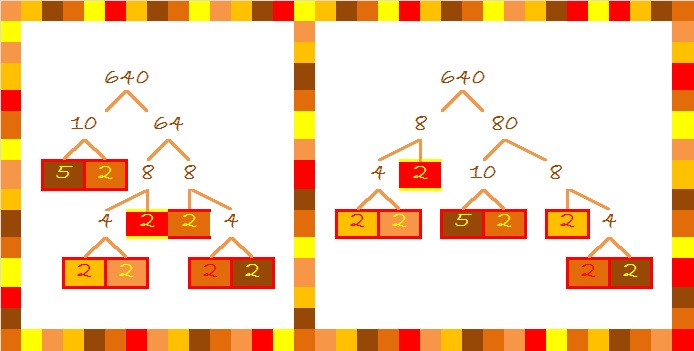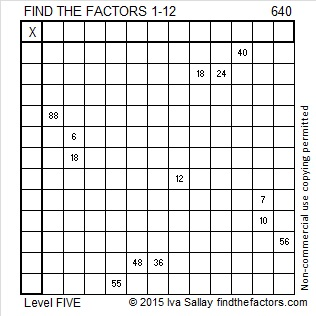# 640 Fall Factor Trees and Level 5

Because it is fall, and 640 has many factors, I decided to make factor trees using fall colors. Get out your rakes!There are many other possible factor trees for 640, but raking leaves can be a lot of work, so I only made two of them.

640 is the hypotenuse of the Pythagorean triple 384-512-640.

OEIS.org informs us that 640 = 16!!!!!!, but if you type 16!!!!!! into a calculator, you will get an error message as soon as you type !!.

16!!!!!! ≠ (((((16!)!)!)!)!)!

There are 6 !’s so 16!!!!!! = 16(16-6)(16-12) = 16 x 10 x 4.

Here is this week’s Level 5 puzzle:Print the puzzles or type the solution on this excel file: 12 Factors 2015-10-05

—————————————————————————————————

• 640 is a composite number.
• Prime factorization: 640 = 2 x 2 x 2 x 2 x 2 x 2 x 2 x 5, which can be written 640 = (2^7) x 5
• The exponents in the prime factorization are 7 and 1. Adding one to each and multiplying we get (7 + 1)(1 + 1) = 8 x 2 = 16. Therefore 640 has exactly 16 factors.
• Factors of 640: 1, 2, 4, 5, 8, 10, 16, 20, 32, 40, 64, 80, 128, 160, 320, 640
• Factor pairs: 640 = 1 x 640, 2 x 320, 4 x 160, 5 x 128, 8 x 80, 10 x 64, 16 x 40, or 20 x 32
• Taking the factor pair with the largest square number factor, we get √640 = (√64)(√10) = 8√10 ≈ 25.298221—————————————————————————————————